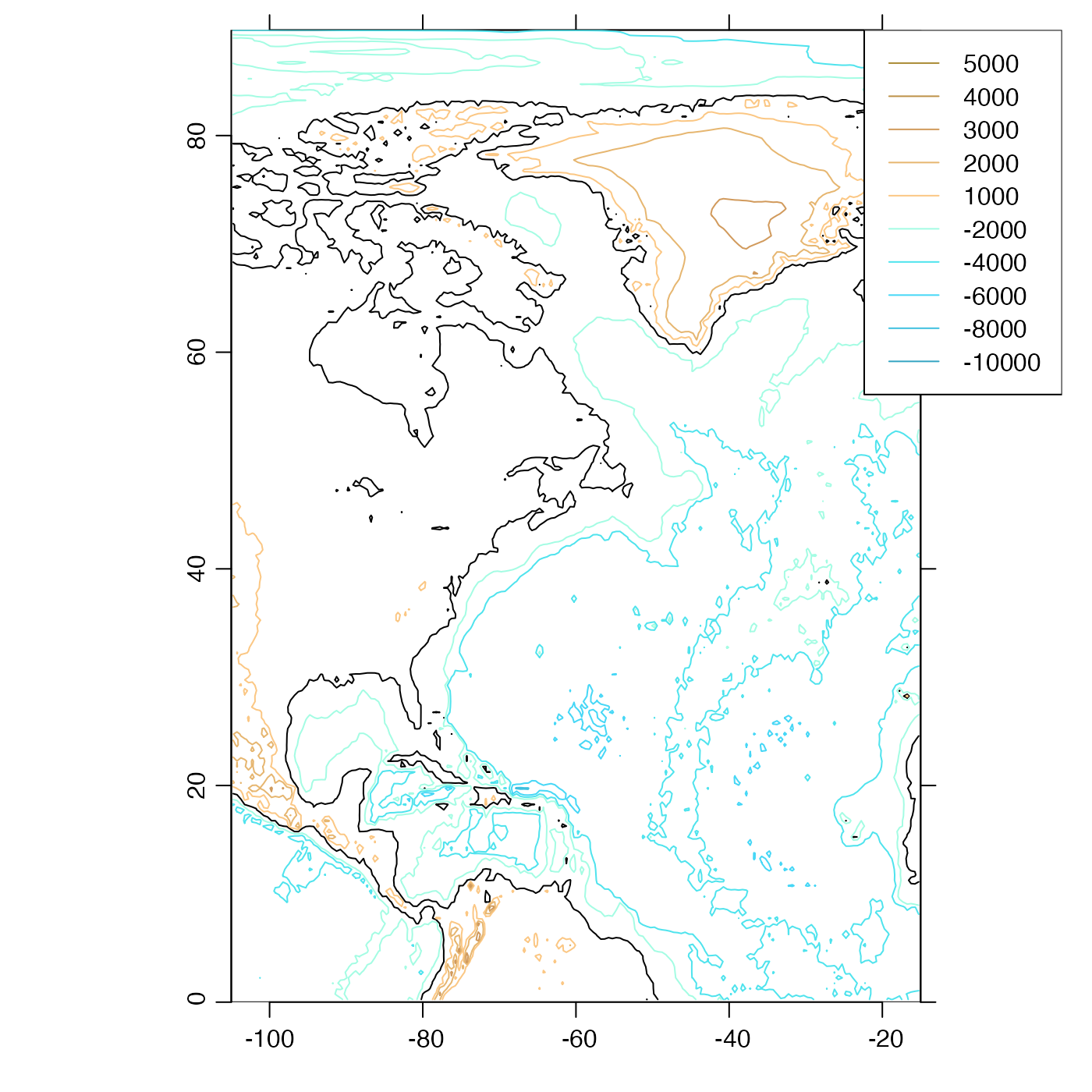This plots contours of topographic elevation. The plot aspect ratio is set based on the middle latitude in the plot. The line properties, such as land.lwd, may either be a single item, or a vector; in the latter case, the length must match the length of the corresponding properties, e.g. land.z.

# S4 method for topo
plot(
x,
xlab = "",
ylab = "",
asp,
clongitude,
clatitude,
span,
expand = 1.5,
water.z,
col.water,
lty.water,
lwd.water,
land.z,
col.land,
lty.land,
lwd.land,
geographical = FALSE,
location = "topright",
mgp = getOption("oceMgp"),
mar = c(mgp + 1, mgp + 1, 1, 1),
debug = getOption("oceDebug"),
...
)

## Arguments

x

a topo object.

xlab, ylab

Character strings giving a label for the x and y axes.

asp

Aspect ratio for plot. The default is for plot.coastline to set the aspect ratio to give natural latitude-longitude scaling somewhere near the centre latitude on the plot. Often, it makes sense to set asp yourself, e.g. to get correct shapes at 45N, use asp=1/cos(45*pi/180). Note that the land mass is not symmetric about the equator, so to get good world views you should set asp=1 or set ylim to be symmetric about zero. Any given value of asp is ignored, if clongitude and clatitude are given.

clongitude

Optional center longitude of map, in degrees east; see clatitude.

clatitude

Optional center latitude of map, in degrees north. If this and clongitude are provided, then any provided value of asp is ignored, and instead the plot aspect ratio is computed based on the center latitude. Also, if clongitude and clatitude are provided, then span must be, also.

span

Optional suggested span of plot, in kilometers (must be supplied, if clongitude and clatitude are supplied).

expand

Numerical factor for the expansion of plot limits, showing area outside the plot, e.g. if showing a ship track as a coastline, and then an actual coastline to show the ocean boundary. The value of expand is ignored if either xlim or ylim is given.

water.z

Depths at which to plot water contours. If not provided, these are inferred from the data.

col.water

Colors corresponding to water.z values. If not provided, these will be "fill" colors from oce.colorsGebco().

lty.water

Line type(s) for water contours.

lwd.water

Line width(s) for water contours.

land.z

Depths at which to plot land contours. If not provided, these are inferred from the data. If set to NULL, no land contours will be plotted.

col.land

Colors corresponding to land.z values. If not provided, these will be "fill" colors from oce.colorsGebco().

lty.land

Line type(s) for land contours.

lwd.land

Line width(s) for land contours.

geographical

Logical, indicating whether to plot latitudes and longitudes without minus signs.

location

Location for a legend (or "none", for no legend).

mgp

3-element numerical vector to use for par(mgp), and also for par(mar), computed from this. The default is tighter than the R default, in order to use more space for the data and less for the axes.

mar

Four-element numerical vector to be used with par("mar").

debug

Numerical value, with positive values indicating higher levels of debugging.

...

Additional arguments passed on to plotting functions.

Other functions that plot oce data: download.amsr(), plot,adp-method, plot,adv-method, plot,amsr-method, plot,argo-method, plot,bremen-method, plot,cm-method, plot,coastline-method, plot,ctd-method, plot,gps-method, plot,ladp-method, plot,landsat-method, plot,lisst-method, plot,lobo-method, plot,met-method, plot,odf-method, plot,rsk-method, plot,satellite-method, plot,sealevel-method, plot,section-method, plot,tidem-method, plot,windrose-method, plot,xbt-method, plotProfile(), plotScan(), plotTS(), tidem-class

Other things related to topo data: [[,topo-method, [[<-,topo-method, as.topo(), download.topo(), read.topo(), subset,topo-method, summary,topo-method, topo-class, topoInterpolate(), topoWorld

Dan Kelley

## Examples

library(oce)
data(topoWorld)
plot(topoWorld, clongitude=-60, clatitude=45, span=10000)